Fits generalized linear latent variable model for multivariate data. The model can be fitted using Laplace approximation method or variational approximation method.

gllvm(
y = NULL,
X = NULL,
TR = NULL,
data = NULL,
formula = NULL,
lv.formula = NULL,
num.lv = NULL,
num.lv.c = 0,
num.RR = 0,
family,
row.eff = FALSE,
offset = NULL,
sd.errors = TRUE,
method = "VA",
randomX = NULL,
dependent.row = FALSE,
beta0com = FALSE,
zeta.struc = "species",
plot = FALSE,
dist = 0,
corWithin = FALSE,
Power = 1.1,
seed = NULL,
scale.X = TRUE,
return.terms = TRUE,
control = list(reltol = 1e-10, TMB = TRUE, optimizer = "optim", max.iter = 2000,
maxit = 4000, trace = FALSE, optim.method = NULL),
control.va = list(Lambda.struc = "unstructured", Ab.struct = "unstructured",
diag.iter = 1, Ab.diag.iter = 0, Lambda.start = c(0.3, 0.3, 0.3)),
control.start = list(starting.val = "res", n.init = 1, jitter.var = 0, start.fit =
NULL, start.lvs = NULL, randomX.start = "zero", quad.start = 0.01, start.struc =
"LV"),
...
)

## Arguments

y (n x m) matrix of responses. matrix or data.frame of environmental covariates. matrix or data.frame of trait covariates. data in long format, that is, matrix of responses, environmental and trait covariates and row index named as "id". When used, model needs to be defined using formula. This is alternative data input for y, X and TR. an object of class "formula" (or one that can be coerced to that class): a symbolic description of the model to be fitted (for fixed-effects predictors). an object of class "formula" (or one that can be coerced to that class): a symbolic description of the model to be fitted (for latent variables). number of latent variables, d, in gllvm model. Non-negative integer, less than number of response variables (m). Defaults to 0. number of latent variables, d, in gllvm model to constrain, with residual term. Non-negative integer, less than number of response (m) and equal to, or less than, the number of predictor variables (k). Defaults to 0. Requires specification of "lv.formula" in combination with "X" or "datayx". Can be used in combination with num.lv and fixed-effects, but not with traits. number of latent variables, d, in gllvm model to constrain, without residual term (reduced rank regression). Cannot yet be combined with traits. distribution function for responses. Options are "negative.binomial" (with log link), poisson(link = "log"), binomial(link = "probit") (and also with link = "logit" when method = "LA" or method = "EVA"), zero inflated poisson ("ZIP"), gaussian(link = "identity"), Tweedie ("tweedie") (with log link, for "LA" and "EVA"-method), "gamma" (with log link), "exponential" (with log link), beta ("beta") (with logit and probit link, for "LA" and "EVA"-method) and "ordinal" (only with "VA"-method). FALSE, fixed, "random" or formula to define the structure for the row parameters. Indicating whether row effects are included in the model as a fixed or as a random effects. Defaults to FALSE when row effects are not included. Structured random row effects can be defined via formula, eg. ~(1|groups), when unique row effects are set for each group, not for all rows, grouping variable need to be included in X. Correlation structure between random group effects/intercepts can also be set using ~struc(1|groups), where option to 'struc' are corAR1 (AR(1) covariance), corExp (exponentielly decaying, see argument 'dist') and corCS (compound symmetry). Correlation structure can be set between or within groups, see argument 'corWithin'. vector or matrix of offset terms. either FALSE(default), TRUE, or LV. If FALSE models species responses as a linear function of the latent variables. If TRUE models species responses as a quadratic function of the latent variables. If LV assumes species all have the same quadratic coefficient per latent variable. logical. If TRUE (default) standard errors for parameter estimates are calculated. model can be fitted using Laplace approximation method (method = "LA") or variational approximation method (method = "VA"), or with extended variational approximation method (method = "EVA") when VA is not applicable. If particular model has not been implemented using the selected method, model is fitted using the alternative method as a default. Defaults to "VA". formula for species specific random effects of environmental variables in fourth corner model. Defaults to NULL, when random slopes are not included. logical, whether or not random row effects are correlated (dependent) with the latent variables. Defaults to FALSE when correlation terms are not included. logical, if FALSE column-specific intercepts are assumed. If TRUE, a common intercept is used which is allowed only for fourth corner models. Structure for cut-offs in the ordinal model. Either "common", for the same cut-offs for all species, or "species" for species-specific cut-offs. For the latter, classes are arbitrary per species, each category per species needs to have at least one observations. Defaults to "species". logical, if TRUE ordination plots will be printed in each iteration step when TMB = FALSE. Defaults to FALSE. link function for binomial family if method = "LA" and beta family. Options are "logit" and "probit. coordinates or time points used for row parameters correlation structure corExp. logical. If TRUE, correlation is set between row effects of the observation units within group. Correlation and groups can be defined using row.eff. Defaults to FALSE, when correlation is set for row parameters between groups. fixed power parameter in Tweedie model. Scalar from interval (1,2). Defaults to 1.1. a single seed value, defaults to NULL. if TRUE, covariates are scaled when fourth corner model is fitted. logical, if TRUE 'terms' object is returned. logical, if TRUE gradients are checked for large values (>0.01) even if the optimization algorithm did converge. A list with the following arguments controlling the optimization: reltol: convergence criteria for log-likelihood, defaults to 1e-8. TMB: logical, if TRUE model will be fitted using Template Model Builder (TMB). TMB is always used if method = "LA". Defaults to TRUE. optimizer: if TMB=TRUE, log-likelihood can be optimized using "optim" (default) or "nlminb". max.iter: maximum number of iterations when TMB = FALSE or for optimizer = "nlminb" when TMB = TRUE, defaults to 200. maxit: maximum number of iterations for optimizer, defaults to 4000. trace: logical, if TRUE in each iteration step information on current step will be printed. Defaults to FALSE. Only with TMB = FALSE. optim.method: optimization method to be used if optimizer is "optim". Defaults to "BFGS", and "L-BFGS-B" to Tweedie family due the limited-memory use. A list with the following arguments controlling the variational approximation method: Lambda.struc: covariance structure of VA distributions for latent variables when method = "VA", "unstructured" or "diagonal". Ab.struct: covariance structure of VA distributions for random slopes when method = "VA", "unstructured" or "diagonal". diag.iter: non-negative integer which can sometimes be used to speed up the updating of variational (covariance) parameters in VA method. Can sometimes improve the accuracy. If TMB = TRUE either 0 or 1. Defaults to 1. Ab.diag.iter: As above, but for variational covariance of random slopes. Lambda.start: starting values for variances in VA distributions for latent variables, random row effects and random slopes in variational approximation method. Defaults to 0.2. A list with the following arguments controlling the starting values: starting.val: starting values can be generated by fitting model without latent variables, and applying factorial analysis to residuals to get starting values for latent variables and their coefficients (starting.val = "res"). Another options are to use zeros as a starting values (starting.val = "zero") or initialize starting values for latent variables with (n x num.lv) matrix. Defaults to "res", which is recommended. n.init: number of initial runs. Uses multiple runs and picks up the one giving highest log-likelihood value. Defaults to 1. start.fit: object of class 'gllvm' which can be given as starting parameters for count data (poisson, NB, or ZIP). start.lvs: initialize starting values for latent variables with (n x num.lv) matrix. Defaults to NULL. jitter.var: jitter variance for starting values of latent variables. Defaults to 0, meaning no jittering. randomX.start: Starting value method for the random slopes. Options are "zero" and "res". Defaults to "zero". start.struc: Starting value method for the quadratic term. Options are "LV" (default) and "all". quad.start: Starting values for quadratic coefficients. Defaults to 0.01. Not used.

## Value

An object of class "gllvm" includes the following components:

call

function call

logL

log likelihood

lvs

latent variables

params

list of parameters

• theta coefficients related to latent variables

• LvXcoef Covariate coefficients related to constrained latent variables

• beta0 column specific intercepts

• Xcoef coefficients related to environmental covariates X

• B coefficients in fourth corner model

• row.params row-specific intercepts

• phi dispersion parameters $$\phi$$ for negative binomial or Tweedie family, probability of zero inflation for ZIP family, standard deviation for gaussian family or shape parameter for gamma family

• inv.phi dispersion parameters $$1/\phi$$ for negative binomial

Power

power parameter $$\nu$$ for Tweedie family

sd

list of standard errors of parameters

prediction.errors

list of prediction covariances for latent variables and variances for random row effects when method "LA" is used

A, Ar

covariance matrices for variational densities of latent variables and variances for random row effects

## Details

Fits generalized linear latent variable models as in Hui et al. (2015 and 2017) and Niku et al. (2017). Method can be used with two types of latent variable models depending on covariates. If only site related environmental covariates are used, the expectation of response $$Y_{ij}$$ is determined by

$$g(\mu_{ij}) = \eta_{ij} = \alpha_i + \beta_{0j} + x_i'\beta_j + u_i'\theta_j,$$

where $$g(.)$$ is a known link function, $$u_i$$ are $$d$$-variate latent variables ($$d$$<<$$m$$), $$\alpha_i$$ is an optional row effect at site $$i$$, and it can be fixed or random effect (also other structures are possible, see below), $$\beta_{0j}$$ is an intercept term for species $$j$$, $$\beta_j$$ and $$\theta_j$$ are column specific coefficients related to covariates and the latent variables, respectively.

Alternatively, a more complex version of the model can be fitted with quadratic = TRUE, where species are modeled as a quadratic function of the latent variables: $$g(\mu_{ij}) = \eta_{ij} = \alpha_i + \beta_{0j} + x_i'\beta_j + u_i'\theta_j - u_i' D_j u_i$$. Here, D_j is a diagonal matrix of positive only quadratic coefficients, so that the model generates concave shapes only. This implementation follows the ecological theoretical model where species are generally recognized to exhibit non-linear response curves. For a model with quadratic responses, quadratic coefficients are assumed to be the same for all species: $$D_j = D$$. This model requires less information per species and can be expected to be more applicable to most datasets. The quadratic coefficients D can be used to calculate the length of ecological gradients. For quadratic responses, it can be useful to provide the latent variables estimated with a GLLVM with linear responses, or estimated with (Detrended) Correspondence Analysis. The latent variables can then be passed to the start.lvs argument inside the control.start list, which in many cases gives good results.

### Constrained ordination

For GLLVMs with both linear and quadratic response model, the latent variable can be constrained to a series of covariates $$x_lv$$:

$$g(\mu_{ij}) = \alpha_i + \beta_{0j} + x_i'\beta_j + (z_i+X_lv\beta_lv)' \gamma_j - (z_i+X_lv\beta_lv)' D_j (z_i+X_lv\beta_lv) + u_i'\theta_j - u_i' D_j u_i ,$$ where $$z_i+X_lv\beta_lv$$ are constrained latent variables, which account for variation that can be explained by some covariates $$X_lv$$ after accounting for the effects of covariates included in the fixed-effects part of the model $$X$$, and $$u_i$$ are unconstrained latent variables that account for any remaining residual variation.

### Fourth corner model

An alternative model is the fourth corner model (Brown et al., 2014, Warton et al., 2015) which will be fitted if also trait covariates are included. The expectation of response $$Y_{ij}$$ is

$$g(\mu_{ij}) = \alpha_i + \beta_{0j} + x_i'(\beta_x + b_j) + TR_j'\beta_t + vec(B)*kronecker(TR_j,X_i) + u_i'\theta_j - u_i'D_ju_i$$

where g(.), $$u_i$$, $$\beta_{0j}$$ and $$\theta_j$$ are defined as above. Vectors $$\beta_x$$ and $$\beta_t$$ are the main effects or coefficients related to environmental and trait covariates, respectively, matrix $$B$$ includes interaction terms. Vectors $$b_j$$ are optional species-specific random slopes for environmental covariates. The interaction/fourth corner terms are optional as well as are the main effects of trait covariates.

### Structured row effects

In addition to the site-specific random effects, $$\alpha_i$$, it is also possible to set arbitrary structure/design for the row effects. That is, assume that observations / rows $$i=1,...,n$$ in the data matrix are from groups $$t=1,...,T$$, so that each row $$i$$ belongs to one of the groups, denote $$G(i) \in \{1,...,T\}$$. Each group $$t$$ has a number of observations $$n_t$$, so that $$\sum_{t=1}^{T} n_t =n$$. Now we can set random intercept for each group $$t$$, (see argument 'row.eff'):

$$g(\mu_{ij}) = \eta_{ij} = \alpha_{G(i)} + \beta_{0j} + x_i'\beta_j + u_i'\theta_j,$$

There is also a possibility to set correlation structure for the random intercepts between groups, so that $$(\alpha_{1},...,\alpha_{T})^\top \sim N(0, \Sigma_r)$$. That might be the case, for example, when the groups are spatially or temporally dependent. Another option is to set row specific random intercepts $$\alpha_i$$, but to set the correlation structure for the observations within groups, (see argument 'corWithin'). That is, we can set $$corr(\alpha_{i},\alpha_{i'}) = C(i,i') \neq 0$$ according to some correlation function $$C$$, when $$G(i)=G(i')$$. This model is restricted to the case, where each group has equal number of observations (rows), that is $$n_t=n_{t'}$$ for all $$t,t' \in \{1,...,T\}$$.

The correlation structures available in the package are

• corAR1 autoregressive process of order 1.

• corExp exponentially decaying, see argument 'dist'.

• corCS compound symmetry.

### Starting values

The method is sensitive for the choices of initial values of the latent variables. Therefore it is recommendable to use multiple runs and pick up the one giving the highest log-likelihood value (see argument 'n.init'). However, sometimes this is computationally too demanding, and default option starting.val = "res" is recommended. For more details on different starting value methods, see Niku et al., (2018).

Models are implemented using TMB (Kristensen et al., 2015) applied to variational approximation (Hui et al., 2017), extended variational approximation (Korhonen et al., 2021) and Laplace approximation (Niku et al., 2017).

With ordinal family response classes must start from 0 or 1.

### Distributions

Mean and variance for distributions are defined as follows.

• For count data family = poisson(): Expectation $$E[Y_{ij}] = \mu_{ij}$$, variance $$V(\mu_{ij}) = \mu_{ij}$$, or

• family = "negative.binomial": Expectation $$E[Y_{ij}] = \mu_{ij}$$, variance $$V(\mu_{ij}) = \mu_{ij}+\mu_{ij}^2\phi_j$$, or

• family = "ZIP": Expectation $$E[Y_{ij}] = (1-p)\mu_{ij}$$, variance $$V(\mu_{ij}) = \mu_{ij}(1-p)(1+\mu_{ij}p)$$.

• For binary data family = binomial(): Expectation $$E[Y_{ij}] = \mu_{ij}$$, variance $$V(\mu_{ij}) = \mu_{ij}(1-\mu_{ij})$$.

• For percent cover data $$0 < Y_{ij} < 1$$ family = "beta": Expectation $$E[Y_{ij}] = \mu_{ij}$$, variance $$V(\mu_{ij}) = \mu_{ij}(1-\mu_{ij})//(1+\phi_j)$$.

• For positive continuous data family = "gamma":Expectation $$E[Y_{ij}] = \mu_{ij}$$, variance $$V(\mu_{ij}) = \mu_{ij}^2/\phi_j$$, where $$\phi_j$$ is species specific shape parameter.

• For non-negative continuous data family = "exponential":Expectation $$E[Y_{ij}] = \mu_{ij}$$, variance $$V(\mu_{ij}) = \mu_{ij}^2$$.

• For non-negative continuous or biomass datafamily = "tweedie" Expectation $$E[Y_{ij}] = \mu_{ij}$$, variance $$V(\mu_{ij}) = \phi_j*\mu_{ij}^\nu$$, where $$\nu$$ is a power parameter of Tweedie distribution. See details Dunn and Smyth (2005).

• For ordinal data family = "ordinal": Cumulative probit model, see Hui et.al. (2016).

• For normal distributed data family = gaussian(): Expectation $$E[Y_{ij}] = \mu_{ij}$$, variance $$V(y_{ij}) = \phi_j^2.$$

## Note

If function gives warning: 'In f(x, order = 0) : value out of range in 'lgamma'', optimizer have visited an area where gradients become too big. It is automatically fixed by trying another step in the optimization process, and can be ignored if errors do not occur.

Brown, A. M., Warton, D. I., Andrew, N. R., Binns, M., Cassis, G., and Gibb, H. (2014). The fourth-corner solution - using predictive models to understand how species traits interact with the environment. Methods in Ecology and Evolution, 5:344-352.

Dunn, P. K. and Smyth, G. K. (2005). Series evaluation of tweedie exponential dispersion model densities. Statistics and Computing, 15:267-280.

Hui, F. K. C., Taskinen, S., Pledger, S., Foster, S. D., and Warton, D. I. (2015). Model-based approaches to unconstrained ordination. Methods in Ecology and Evolution, 6:399-411.

Hui, F. K. C., Warton, D., Ormerod, J., Haapaniemi, V., and Taskinen, S. (2017). Variational approximations for generalized linear latent variable models. Journal of Computational and Graphical Statistics. Journal of Computational and Graphical Statistics, 26:35-43.

Kasper Kristensen, Anders Nielsen, Casper W. Berg, Hans Skaug, Bradley M. Bell (2016). TMB: Automatic Differentiation and Laplace Approximation. Journal of Statistical Software, 70(5), 1-21.

Korhonen, P., Hui, F. K. C., Niku, J., and Taskinen, S. (2021). Fast, universal estimation of latent variable models using extended variational approximations. Submitted.

Niku, J., Warton, D. I., Hui, F. K. C., and Taskinen, S. (2017). Generalized linear latent variable models for multivariate count and biomass data in ecology. Journal of Agricultural, Biological, and Environmental Statistics, 22:498-522.

Niku, J., Brooks, W., Herliansyah, R., Hui, F. K. C., Taskinen, S., and Warton, D. I. (2018). Efficient estimation of generalized linear latent variable models. PLoS One, 14(5):1-20.

Warton, D. I., Guillaume Blanchet, F., O'Hara, R. B., Ovaskainen, O., Taskinen, S., Walker, S. C. and Hui, F. K. C. (2015). So many variables: Joint modeling in community ecology. Trends in Ecology & Evolution, 30:766-779.

coefplot.gllvm, confint.gllvm, ordiplot.gllvm, plot.gllvm, summary.gllvm.

## Author

Jenni Niku <jenni.m.e.niku@jyu.fi>, Wesley Brooks, Riki Herliansyah, Francis K.C. Hui, Sara Taskinen, David I. Warton, Bert van der Veen

## Examples

# Extract subset of the microbial data to be used as an example
data(microbialdata)
X <- microbialdata$Xenv y <- microbialdata$Y[, order(colMeans(microbialdata$Y > 0), decreasing = TRUE)[21:40]] fit <- gllvm(y, X, formula = ~ pH + Phosp, family = poisson()) fit$logL
#>  -4017.074ordiplot(fit)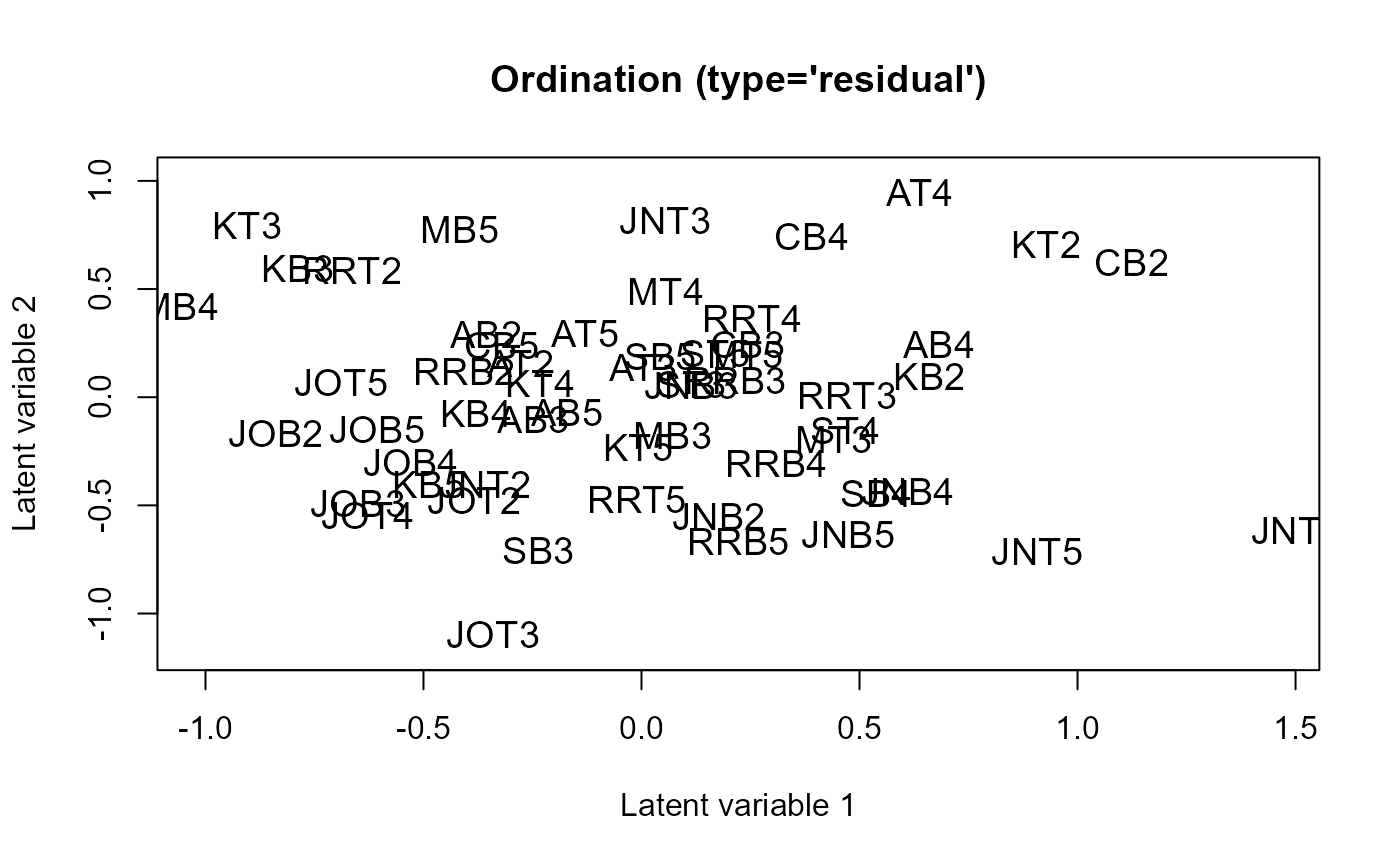coefplot(fit)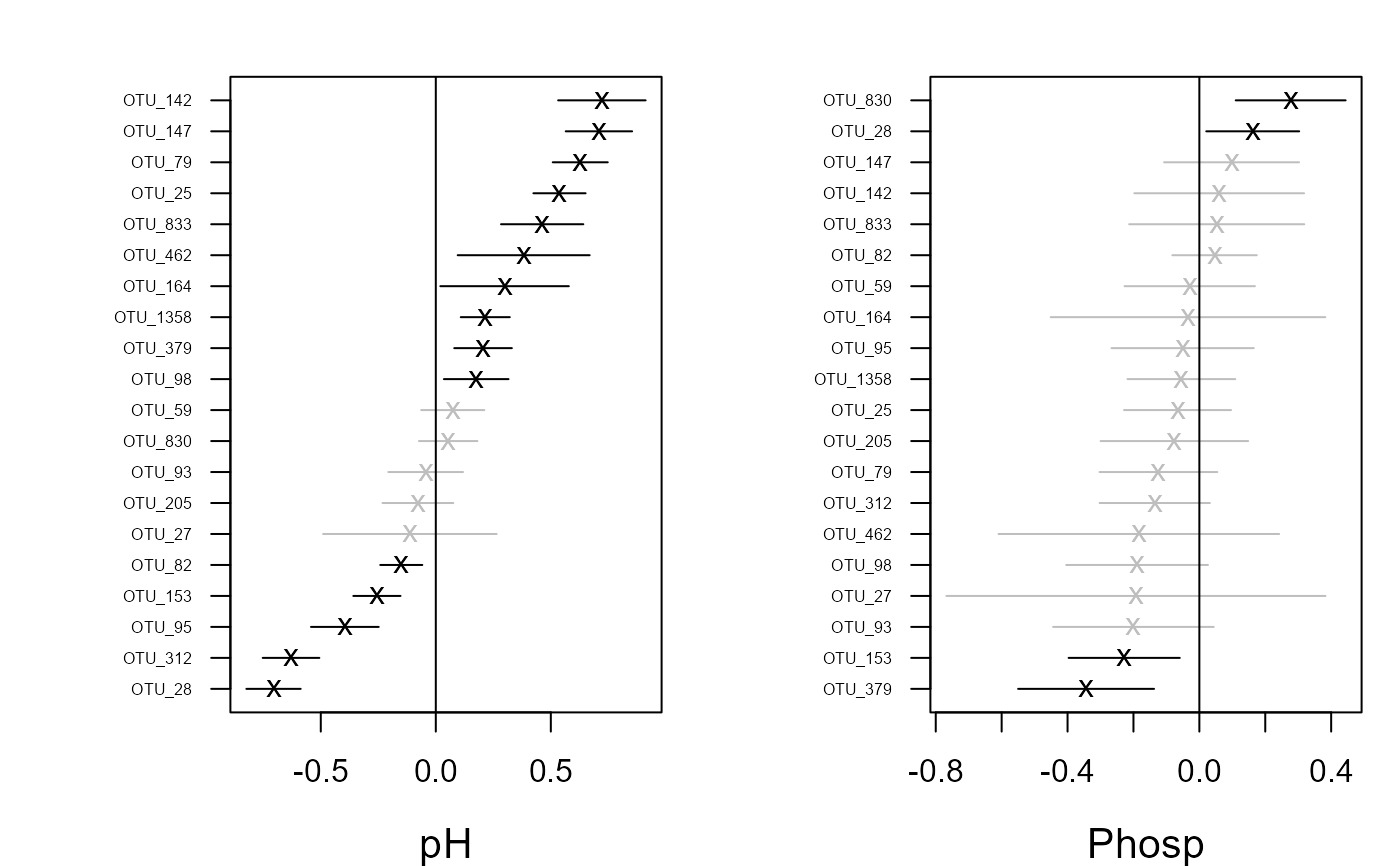# \donttest{
## Load a dataset from the mvabund package
library(mvabund)
data(antTraits)
y <- as.matrix(antTraits$abund) X <- as.matrix(antTraits$env)
TR <- antTraits$traits # Fit model with environmental covariates Bare.ground and Shrub.cover fit <- gllvm(y, X, formula = ~ Bare.ground + Shrub.cover, family = poisson()) ordiplot(fit) coefplot(fit)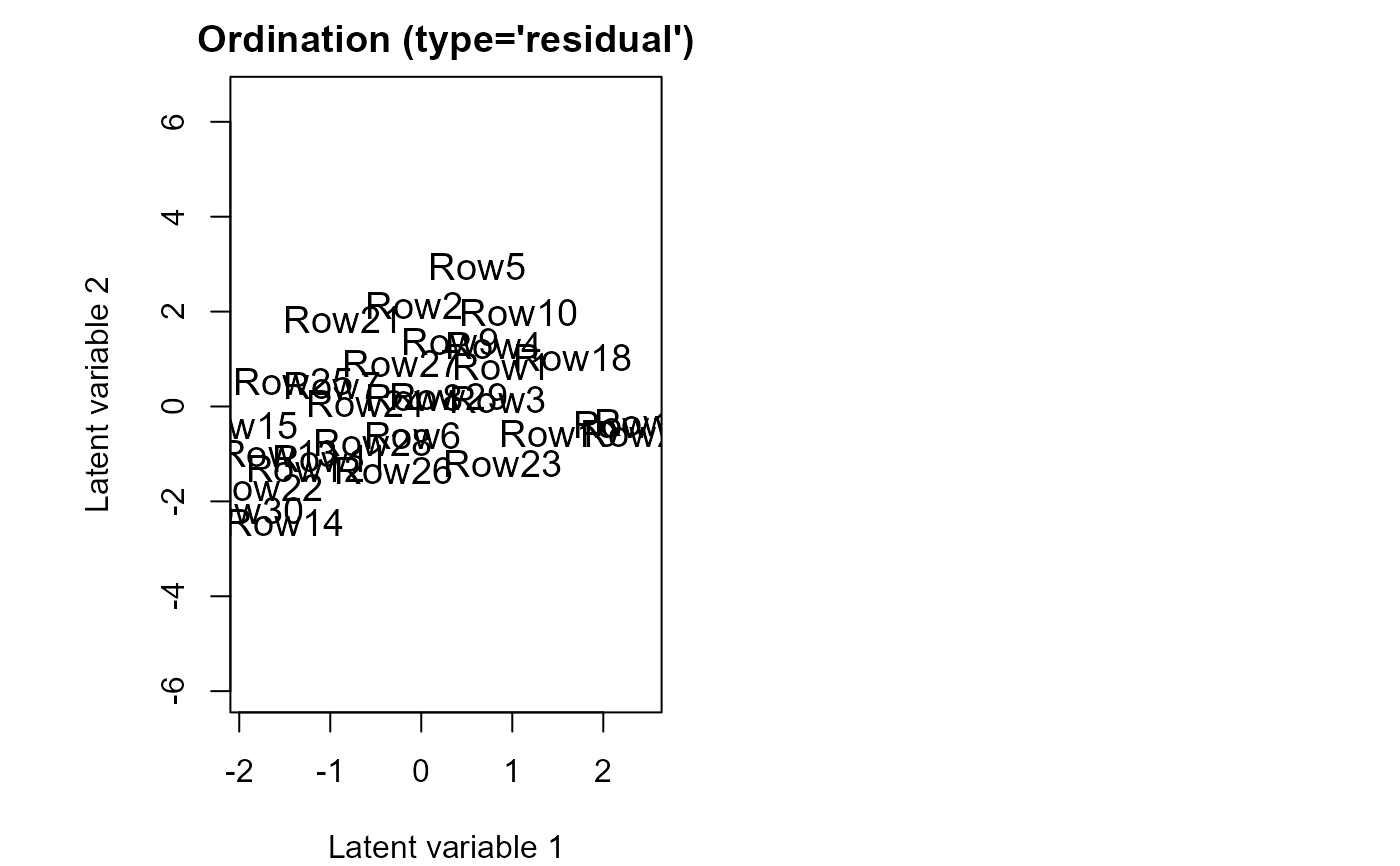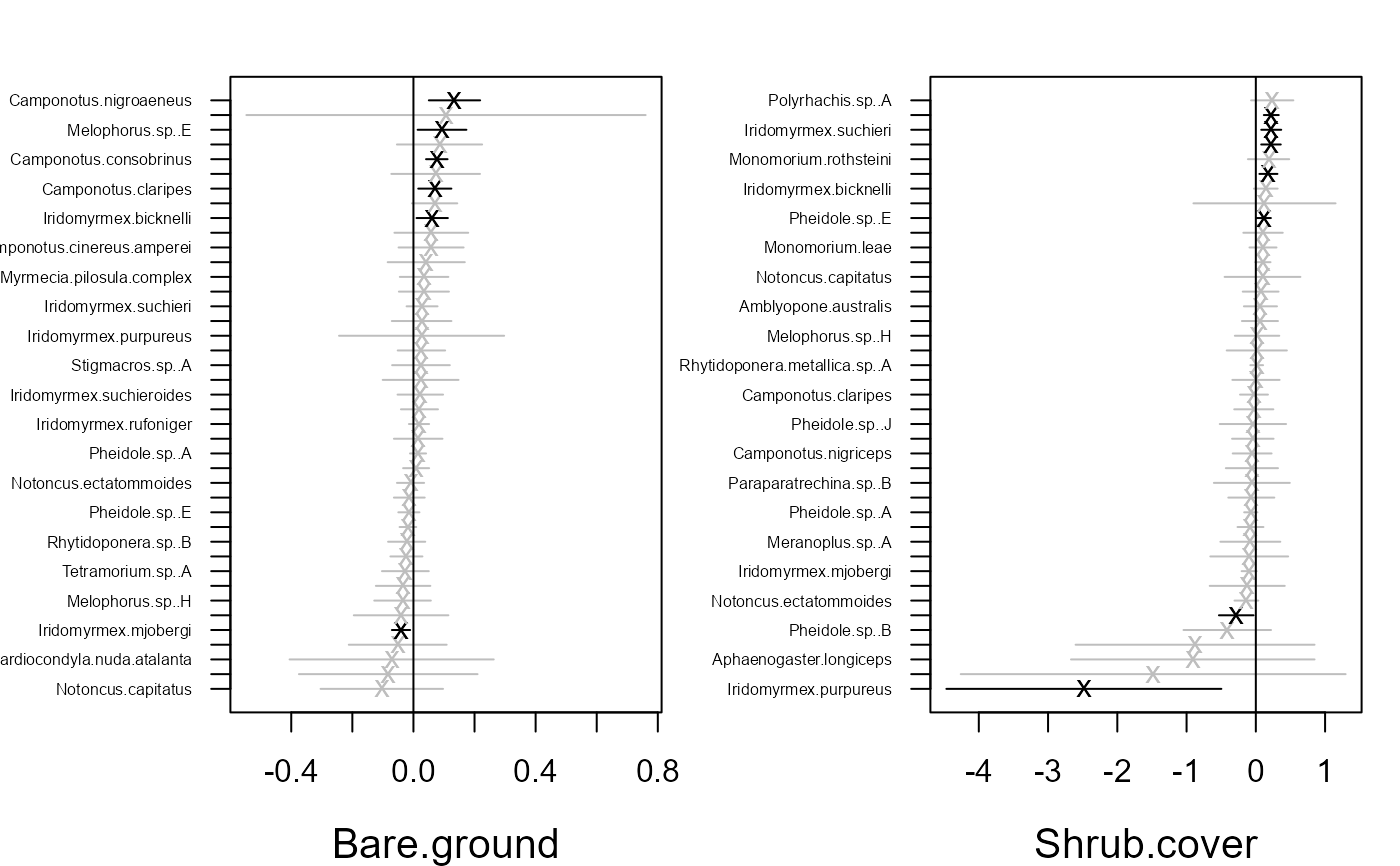## Example 1: Fit model with two unconstrained latent variables # Using variational approximation: fitv0 <- gllvm(y, family = "negative.binomial", method = "VA") ordiplot(fitv0) plot(fitv0, mfrow = c(2,2))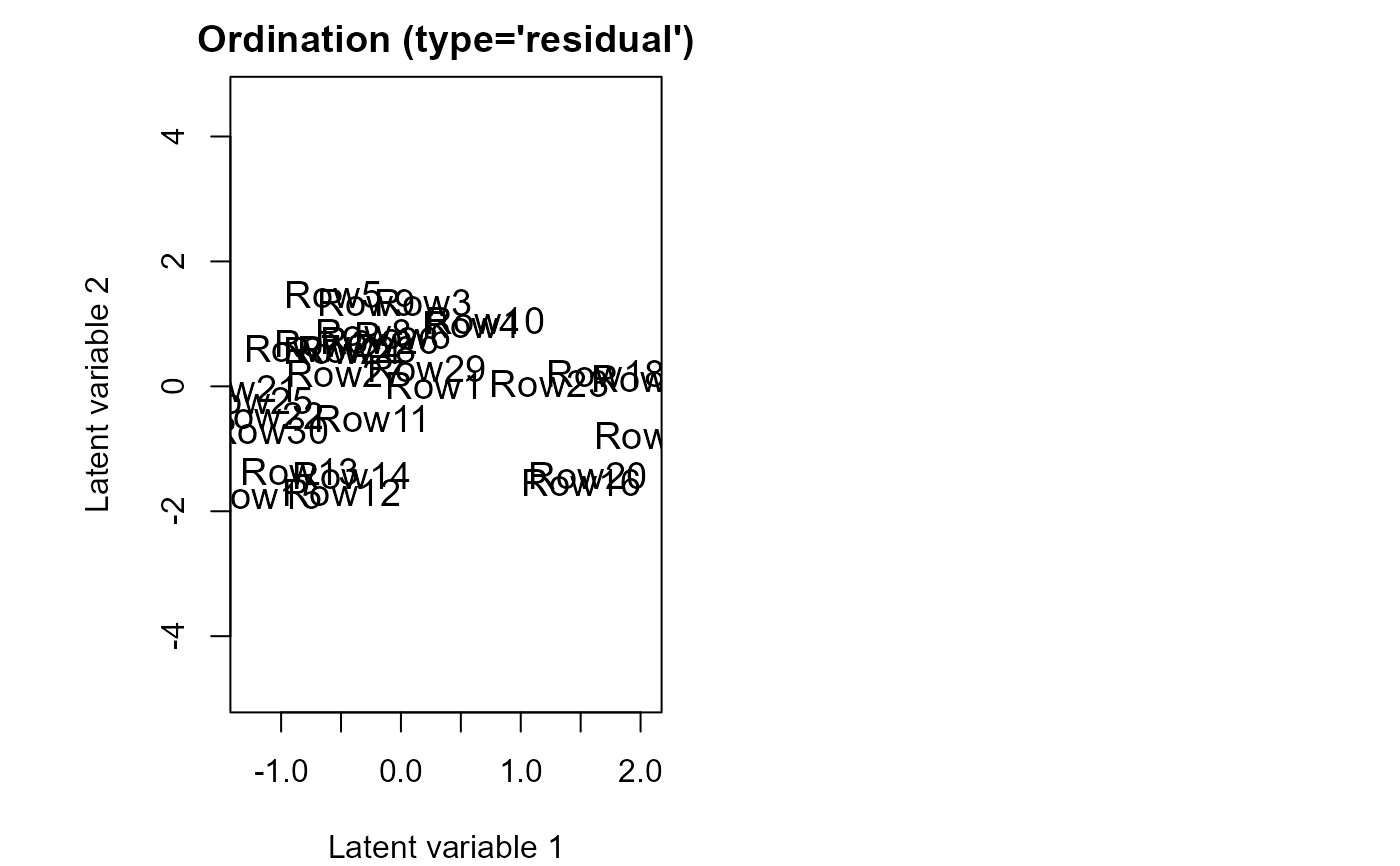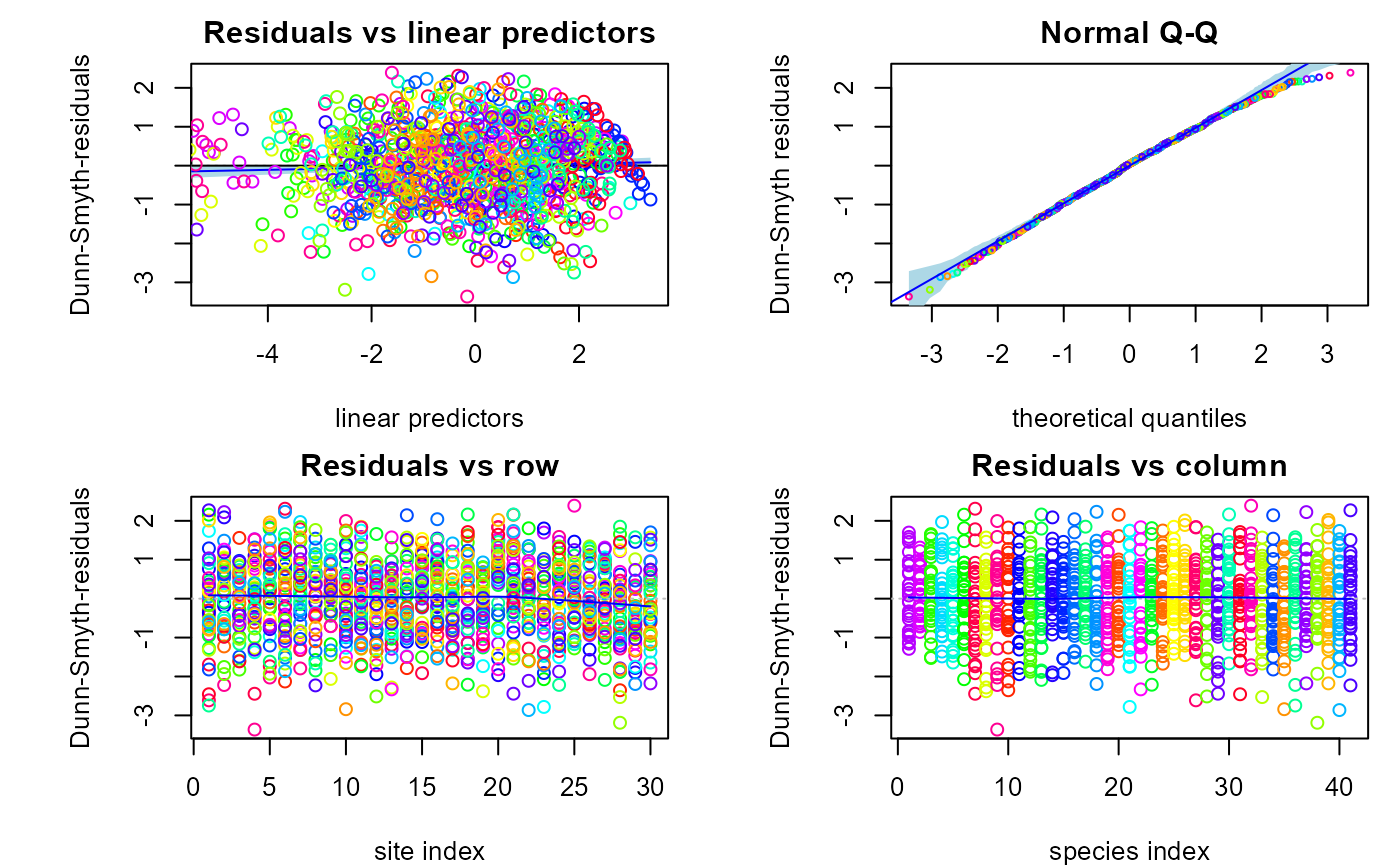summary(fitv0) #> #> Call: #> gllvm(y = y, family = "negative.binomial", method = "VA") #> #> Family: negative.binomial #> #> AIC: 4274.259 AICc: 4324.413 BIC: 4502.654 LL: -1974.1 df: 163 #> #> Constrained LVs: 0 #> Reduced Ranks: 0 #> Unconstrained LVs: 2 #> Standard deviation of LVs: 6.5e-10 4.825e-08 #> #> Formula: ~ 1 #> LV formula: ~0 #> confint(fitv0) #> 2.5 % 97.5 % #> theta.LV1.1 -8.559807e-06 8.561098e-06 #> theta.LV1.2 -1.026828e-04 1.027793e-04 #> theta.LV1.3 1.000000e+00 1.000000e+00 #> theta.LV1.4 -4.049124e+00 -4.049124e+00 #> theta.LV1.5 2.807518e+00 2.807518e+00 #> theta.LV1.6 -4.926051e+00 -4.926051e+00 #> theta.LV1.7 1.064320e+01 1.064320e+01 #> theta.LV1.8 -1.000374e+01 -1.000374e+01 #> theta.LV1.9 -4.084877e-02 -4.084877e-02 #> theta.LV1.10 6.784236e-01 6.784236e-01 #> theta.LV1.11 2.153037e+00 2.153037e+00 #> theta.LV1.12 -2.676853e+00 -2.676853e+00 #> theta.LV1.13 7.948640e+00 7.948640e+00 #> theta.LV1.14 5.974534e+00 5.974534e+00 #> theta.LV1.15 -3.502991e-01 -3.502991e-01 #> theta.LV1.16 -1.248235e-02 -1.248235e-02 #> theta.LV1.17 -2.016163e+00 -2.016163e+00 #> theta.LV1.18 2.026289e+00 2.026289e+00 #> theta.LV1.19 2.283431e+00 2.283431e+00 #> theta.LV1.20 3.418918e+00 3.418918e+00 #> theta.LV1.21 -5.060265e-01 -5.060265e-01 #> theta.LV1.22 -3.557660e+00 -3.557660e+00 #> theta.LV1.23 -2.971996e+00 -2.971996e+00 #> theta.LV1.24 -5.165864e+00 -5.165864e+00 #> theta.LV1.25 2.008130e+00 2.008130e+00 #> theta.LV1.26 -2.235537e+00 -2.235537e+00 #> theta.LV1.27 3.404412e+00 3.404412e+00 #> theta.LV1.28 -2.181165e+00 -2.181165e+00 #> theta.LV1.29 -1.146442e-01 -1.146442e-01 #> theta.LV1.30 2.274720e+00 2.274720e+00 #> theta.LV1.31 1.729655e+00 1.729655e+00 #> theta.LV1.32 -4.184873e+00 -4.184873e+00 #> theta.LV1.33 -1.254221e-01 -1.254221e-01 #> theta.LV1.34 -7.674315e+00 -7.674315e+00 #> theta.LV1.35 1.280614e+00 1.280614e+00 #> theta.LV1.36 2.350545e+00 2.350545e+00 #> theta.LV1.37 -4.107431e+01 -4.107431e+01 #> theta.LV1.38 3.887665e+00 3.887665e+00 #> theta.LV1.39 -1.955253e+00 -1.955253e+00 #> theta.LV1.40 2.187698e+01 2.187698e+01 #> theta.LV1.41 5.880157e+00 5.880157e+00 #> theta.LV2.1 -1.359613e+00 -1.359613e+00 #> theta.LV2.2 -1.237633e+00 -1.237633e+00 #> theta.LV2.3 0.000000e+00 0.000000e+00 #> theta.LV2.4 1.000000e+00 1.000000e+00 #> theta.LV2.5 -7.466637e-02 -7.466637e-02 #> theta.LV2.6 2.246031e+00 2.246031e+00 #> theta.LV2.7 -6.454284e+00 -6.454284e+00 #> theta.LV2.8 1.359185e+00 1.359185e+00 #> theta.LV2.9 2.029447e+00 2.029447e+00 #> theta.LV2.10 5.534417e-01 5.534417e-01 #> theta.LV2.11 4.185678e-01 4.185678e-01 #> theta.LV2.12 -7.654251e+00 -7.654251e+00 #> theta.LV2.13 -5.796581e+00 -5.796581e+00 #> theta.LV2.14 -8.089598e-01 -8.089598e-01 #> theta.LV2.15 9.193673e+00 9.193673e+00 #> theta.LV2.16 3.347865e-01 3.347865e-01 #> theta.LV2.17 -3.970189e+00 -3.970189e+00 #> theta.LV2.18 -5.849696e+00 -5.849696e+00 #> theta.LV2.19 7.843957e-01 7.843957e-01 #> theta.LV2.20 3.093071e-01 3.093071e-01 #> theta.LV2.21 5.972038e+00 5.972038e+00 #> theta.LV2.22 2.370779e+00 2.370779e+00 #> theta.LV2.23 5.857110e-02 5.857110e-02 #> theta.LV2.24 -4.484593e+00 -4.484593e+00 #> theta.LV2.25 -5.956323e-02 -5.956323e-02 #> theta.LV2.26 8.621854e-01 8.621854e-01 #> theta.LV2.27 -7.277528e-01 -7.277528e-01 #> theta.LV2.28 -1.490357e+00 -1.490357e+00 #> theta.LV2.29 9.476559e+00 9.476559e+00 #> theta.LV2.30 2.278312e+00 2.278312e+00 #> theta.LV2.31 -9.016473e-01 -9.016473e-01 #> theta.LV2.32 1.598642e+00 1.598642e+00 #> theta.LV2.33 9.473302e+00 9.473302e+00 #> theta.LV2.34 2.124760e+00 2.124760e+00 #> theta.LV2.35 -9.135984e+00 -9.135984e+00 #> theta.LV2.36 -3.445547e-03 -3.445547e-03 #> theta.LV2.37 5.800343e-01 5.800343e-01 #> theta.LV2.38 4.797402e+00 4.797402e+00 #> theta.LV2.39 2.957744e+00 2.957744e+00 #> theta.LV2.40 6.389521e+00 6.389521e+00 #> theta.LV2.41 -3.517090e+00 -3.517090e+00 #> sigma.LV1 -8.123442e+00 -8.123442e+00 #> sigma.LV2 1.644044e+00 1.644044e+00 #> Intercept.Amblyopone.australis -6.286182e-01 -6.286182e-01 #> Intercept.Aphaenogaster.longiceps 7.096945e-01 7.096945e-01 #> Intercept.Camponotus.cinereus.amperei -1.791786e+00 -1.791786e+00 #> Intercept.Camponotus.claripes 6.592484e-01 6.592484e-01 #> Intercept.Camponotus.consobrinus 1.667812e+00 1.667812e+00 #> Intercept.Camponotus.nigriceps -1.430798e-01 -1.430798e-01 #> Intercept.Camponotus.nigroaeneus -4.054952e-01 -4.054952e-01 #> Intercept.Cardiocondyla.nuda.atalanta -6.931467e-01 -6.931467e-01 #> Intercept.Crematogaster.sp..A -5.679817e-01 -5.679817e-01 #> Intercept.Heteroponera.sp..A 1.402832e+00 1.402832e+00 #> Intercept.Iridomyrmex.bicknelli 1.673985e+00 1.673985e+00 #> Intercept.Iridomyrmex.dromus -5.108332e-01 -5.108332e-01 #> Intercept.Iridomyrmex.mjobergi 1.458568e+00 1.458568e+00 #> Intercept.Iridomyrmex.purpureus 7.096828e-01 7.096828e-01 #> Intercept.Iridomyrmex.rufoniger 2.587790e+00 2.587790e+00 #> Intercept.Iridomyrmex.suchieri 7.576932e-01 7.576932e-01 #> Intercept.Iridomyrmex.suchieroides -1.053560e-01 -1.053560e-01 #> Intercept.Melophorus.sp..E -1.098636e+00 -1.098636e+00 #> Intercept.Melophorus.sp..F 7.577092e-01 7.577092e-01 #> Intercept.Melophorus.sp..H -1.053827e-01 -1.053827e-01 #> Intercept.Meranoplus.sp..A 2.877185e-01 2.877185e-01 #> Intercept.Monomorium.leae 1.554633e+00 1.554633e+00 #> Intercept.Monomorium.rothsteini 1.233553e+00 1.233553e+00 #> Intercept.Monomorium.sydneyense 1.541372e-01 1.541372e-01 #> Intercept.Myrmecia.pilosula.complex -9.162690e-01 -9.162690e-01 #> Intercept.Notoncus.capitatus -4.567467e-01 -4.567467e-01 #> Intercept.Notoncus.ectatommoides 1.064694e+00 1.064694e+00 #> Intercept.Nylanderia.sp..A 1.317301e+00 1.317301e+00 #> Intercept.Ochetellus.glaber -8.362382e-01 -8.362382e-01 #> Intercept.Paraparatrechina.sp..B -3.566995e-01 -3.566995e-01 #> Intercept.Pheidole.sp..A 2.406948e+00 2.406948e+00 #> Intercept.Pheidole.sp..B -5.679852e-01 -5.679852e-01 #> Intercept.Pheidole.sp..E 1.698678e+00 1.698678e+00 #> Intercept.Pheidole.sp..J -1.609472e+00 -1.609472e+00 #> Intercept.Polyrhachis.sp..A -1.204000e+00 -1.204000e+00 #> Intercept.Rhytidoponera.metallica.sp..A 2.116252e+00 2.116252e+00 #> Intercept.Rhytidoponera.sp..B 8.754408e-01 8.754408e-01 #> Intercept.Solenopsis.sp..A -2.014918e+00 -2.014918e+00 #> Intercept.Stigmacros.sp..A -3.393876e-02 -3.393876e-02 #> Intercept.Tapinoma.sp..A 9.295277e-01 9.295277e-01 #> Intercept.Tetramorium.sp..A 6.591983e-01 6.591983e-01 #> inv.phi.Amblyopone.australis 3.463184e-01 3.463184e-01 #> inv.phi.Aphaenogaster.longiceps 3.683457e-02 3.683457e-02 #> inv.phi.Camponotus.cinereus.amperei 5.275887e-01 5.275887e-01 #> inv.phi.Camponotus.claripes 5.898272e-01 5.898272e-01 #> inv.phi.Camponotus.consobrinus 7.243294e-01 7.243294e-01 #> inv.phi.Camponotus.nigriceps 1.400222e-01 1.400222e-01 #> inv.phi.Camponotus.nigroaeneus 4.155421e-01 4.155421e-01 #> inv.phi.Cardiocondyla.nuda.atalanta 6.724015e-02 6.724015e-02 #> inv.phi.Crematogaster.sp..A 9.297578e-02 9.297578e-02 #> inv.phi.Heteroponera.sp..A 8.883178e-01 8.883178e-01 #> inv.phi.Iridomyrmex.bicknelli 7.879137e-01 7.879137e-01 #> inv.phi.Iridomyrmex.dromus 1.900304e-01 1.900304e-01 #> inv.phi.Iridomyrmex.mjobergi 1.258667e+00 1.258667e+00 #> inv.phi.Iridomyrmex.purpureus 1.276568e-01 1.276568e-01 #> inv.phi.Iridomyrmex.rufoniger 1.618122e+00 1.618122e+00 #> inv.phi.Iridomyrmex.suchieri 5.463727e-01 5.463727e-01 #> inv.phi.Iridomyrmex.suchieroides 9.972228e-01 9.972228e-01 #> inv.phi.Melophorus.sp..E 6.196455e-01 6.196455e-01 #> inv.phi.Melophorus.sp..F 1.007397e+00 1.007397e+00 #> inv.phi.Melophorus.sp..H 6.381829e-01 6.381829e-01 #> inv.phi.Meranoplus.sp..A 1.687027e-01 1.687027e-01 #> inv.phi.Monomorium.leae 6.623458e-01 6.623458e-01 #> inv.phi.Monomorium.rothsteini 3.901495e-01 3.901495e-01 #> inv.phi.Monomorium.sydneyense 2.614491e-01 2.614491e-01 #> inv.phi.Myrmecia.pilosula.complex 3.975925e-01 3.975925e-01 #> inv.phi.Notoncus.capitatus 1.269962e-01 1.269962e-01 #> inv.phi.Notoncus.ectatommoides 7.591662e-01 7.591662e-01 #> inv.phi.Nylanderia.sp..A 3.649155e-01 3.649155e-01 #> inv.phi.Ochetellus.glaber 1.924049e-01 1.924049e-01 #> inv.phi.Paraparatrechina.sp..B 5.586946e-02 5.586946e-02 #> inv.phi.Pheidole.sp..A 3.243127e+00 3.243127e+00 #> inv.phi.Pheidole.sp..B 1.914986e-01 1.914986e-01 #> inv.phi.Pheidole.sp..E 1.113459e+00 1.113459e+00 #> inv.phi.Pheidole.sp..J 2.229281e-01 2.229281e-01 #> inv.phi.Polyrhachis.sp..A 9.536347e+05 9.536347e+05 #> inv.phi.Rhytidoponera.metallica.sp..A 2.767528e+00 2.767528e+00 #> inv.phi.Rhytidoponera.sp..B 3.220756e-01 3.220756e-01 #> inv.phi.Solenopsis.sp..A 2.893584e+05 2.893584e+05 #> inv.phi.Stigmacros.sp..A 3.849303e-01 3.849303e-01 #> inv.phi.Tapinoma.sp..A 7.971158e-01 7.971158e-01 #> inv.phi.Tetramorium.sp..A 5.028644e-01 5.028644e-01 ## Example 1a: Fit model with two constrained latent variables and with # quadratic response model # We scale and centre the predictors to improve convergence fity1 <- gllvm(y, X = scale(X), family = "negative.binomial", num.lv.c=2, method="VA") ordiplot(fity1, biplot = TRUE) # Using Laplace approximation: (this line may take about 30 sec to run) fitl0 <- gllvm(y, family = "negative.binomial", method = "LA") ordiplot(fitl0) # Poisson family: fit.p <- gllvm(y, family = poisson(), method = "LA") ordiplot(fit.p)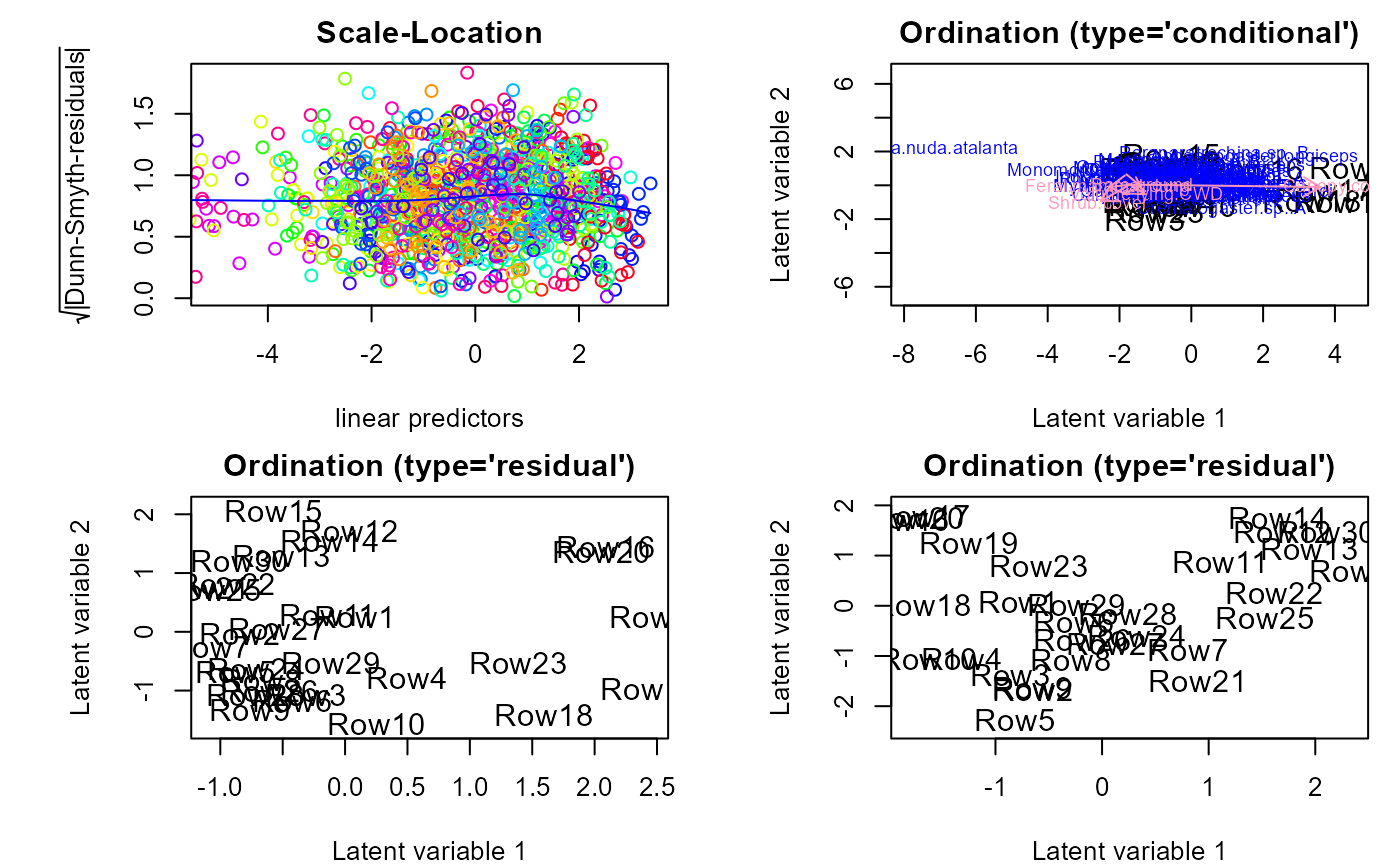# Use poisson model as a starting parameters for ZIP-model, this line # may take few minutes to run fit.z <- gllvm(y, family = "ZIP", method = "LA", control.start = list(start.fit = fit.p)) ordiplot(fit.z) ## Example 2: gllvm with environmental variables # Fit model with two latent variables and all environmental covariates, fitvX <- gllvm(formula = y ~ X, family = "negative.binomial") ordiplot(fitvX, biplot = TRUE) coefplot(fitvX)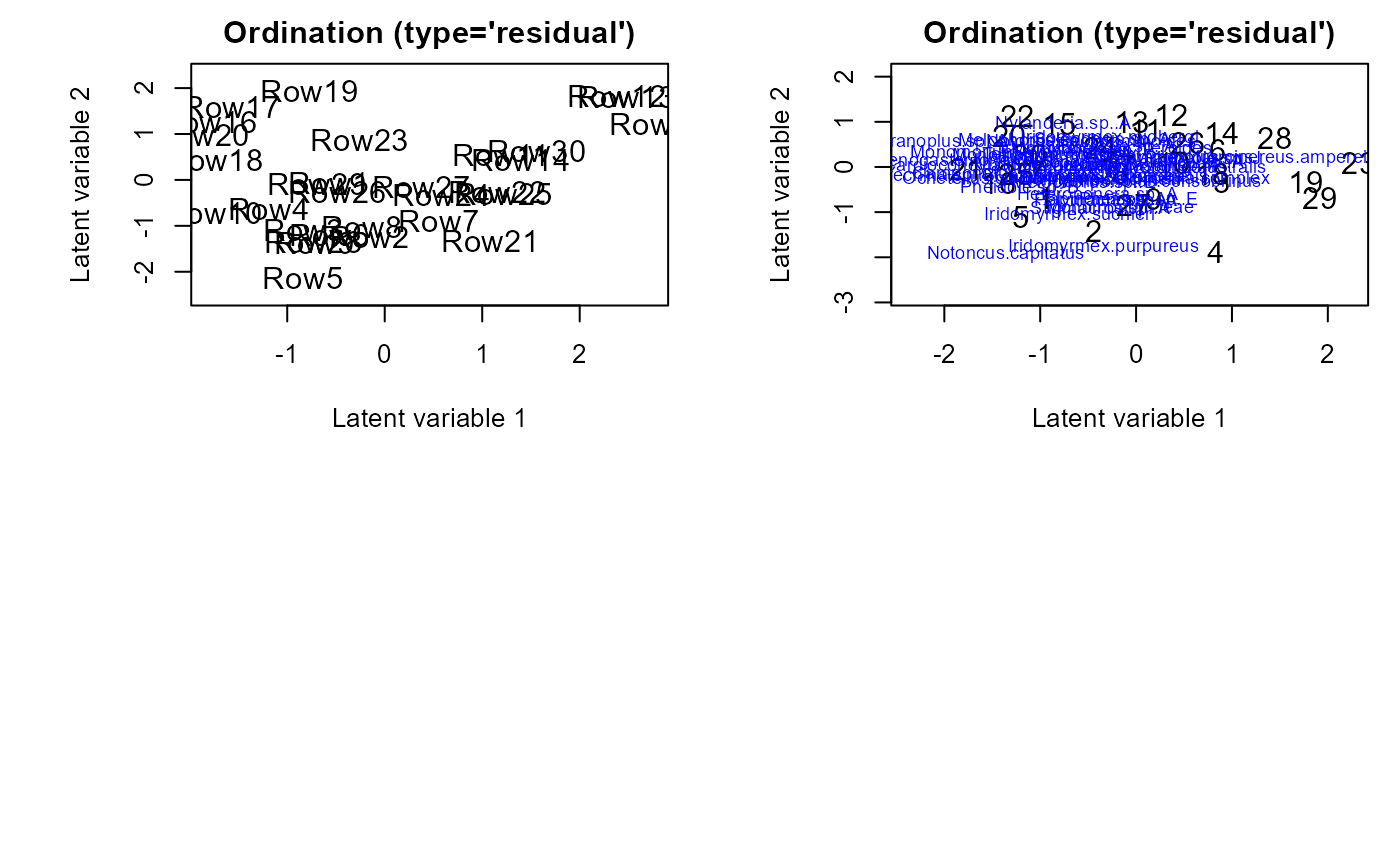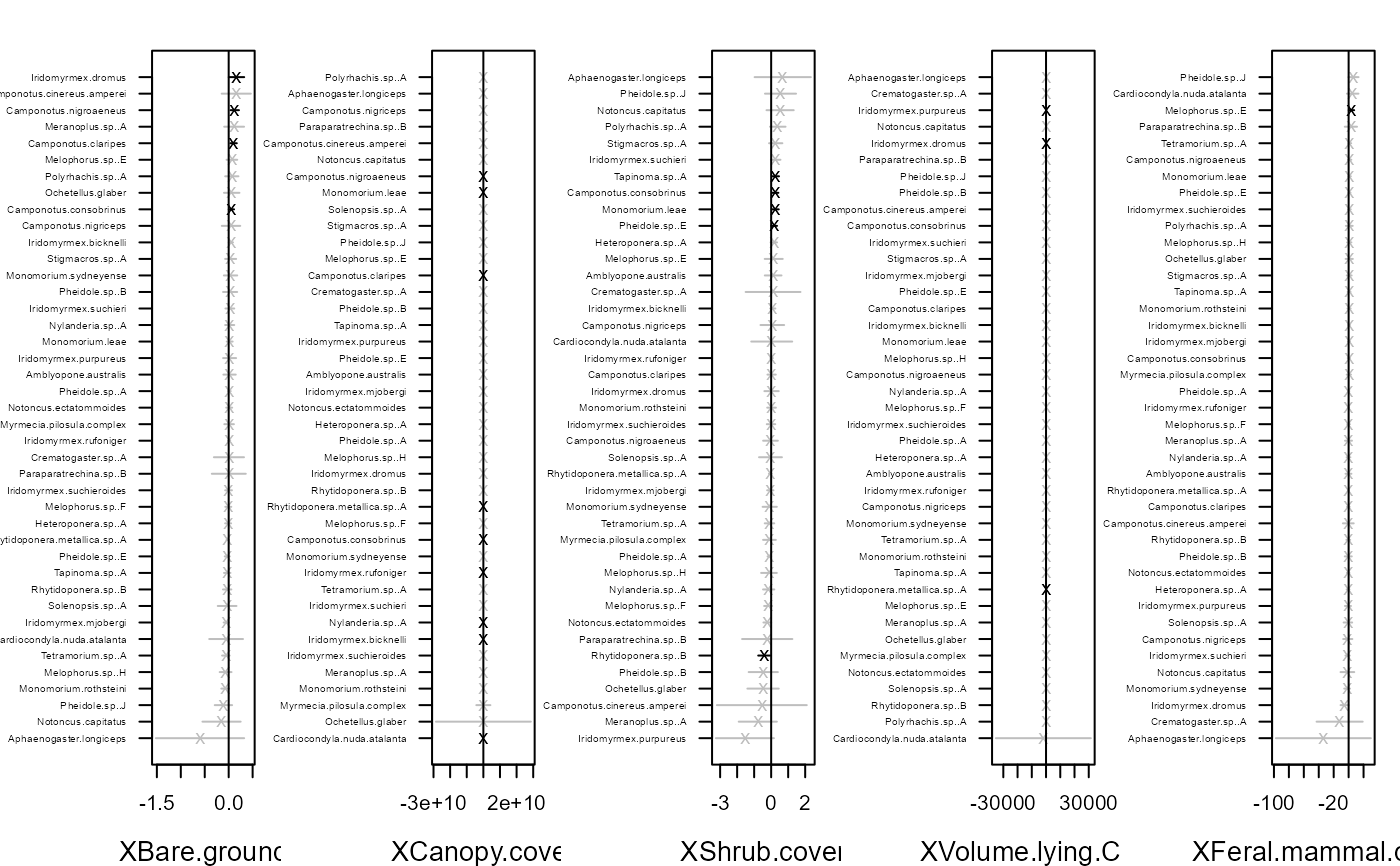# Fit model with environmental covariates Bare.ground and Shrub.cover fitvX2 <- gllvm(y, X, formula = ~ Bare.ground + Shrub.cover, family = "negative.binomial") ordiplot(fitvX2) coefplot(fitvX2)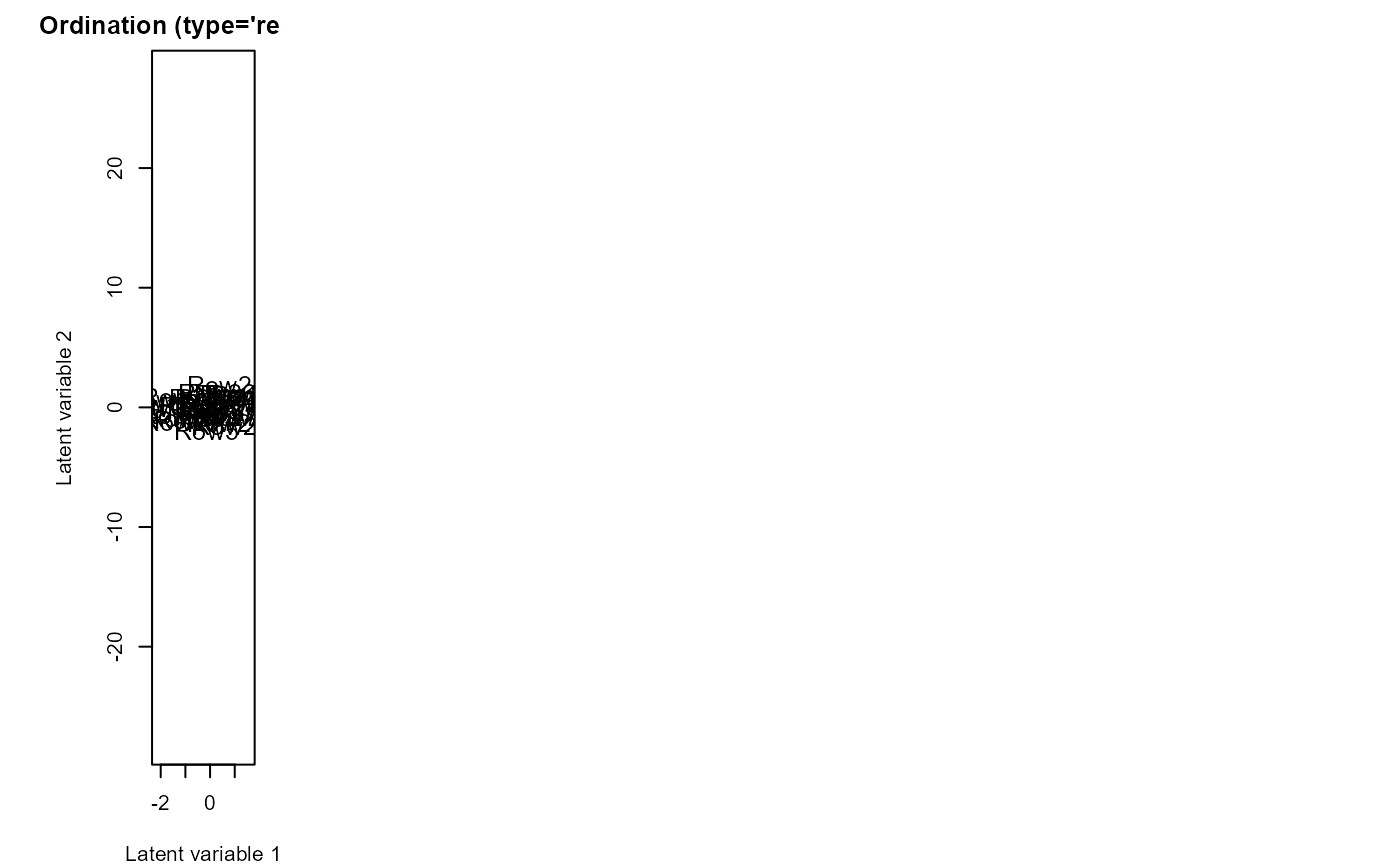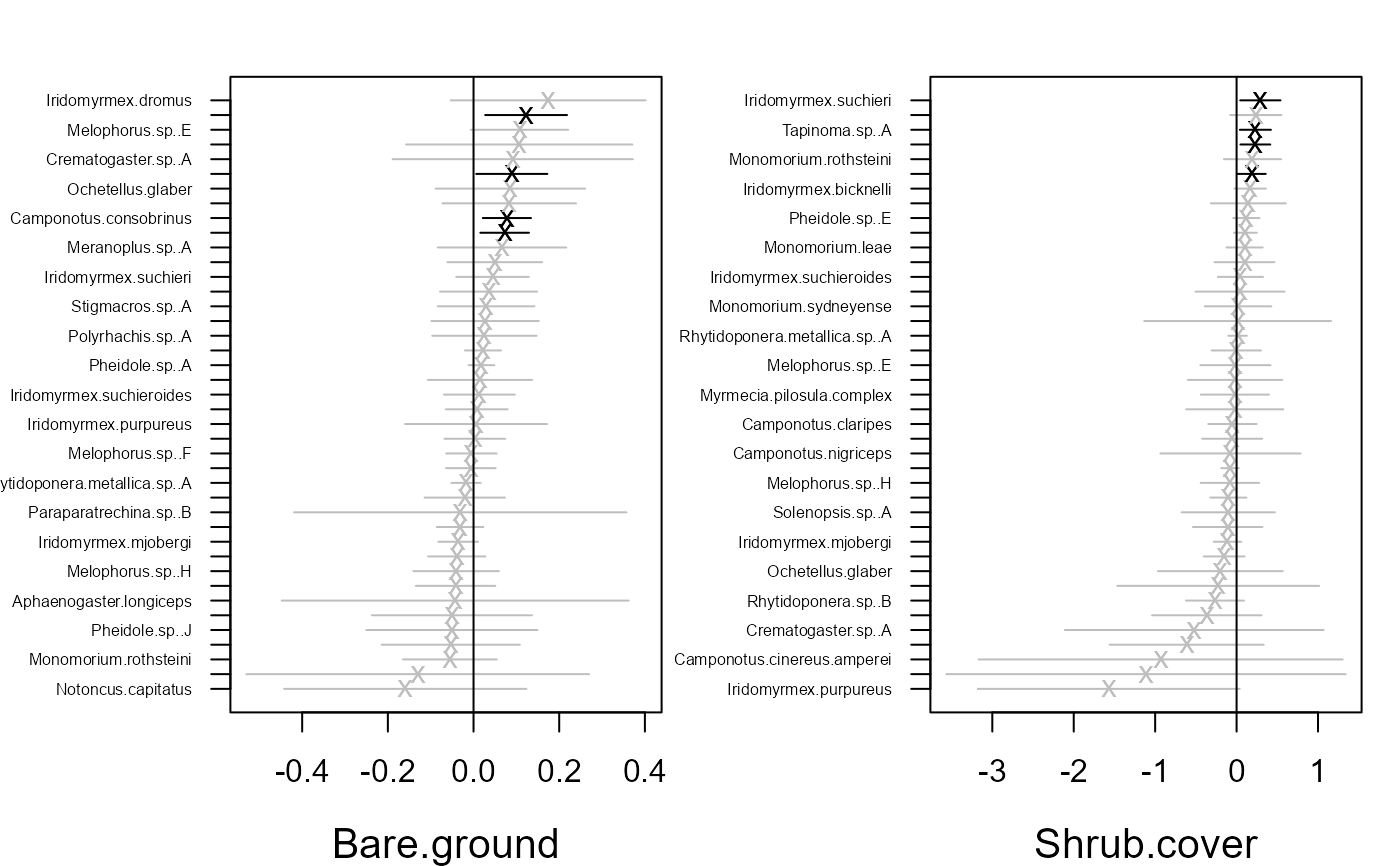# Use 5 initial runs and pick the best one fitvX_5 <- gllvm(y, X, formula = ~ Bare.ground + Shrub.cover, family = "negative.binomial", control.start=list(n.init = 5, jitter.var = 0.1)) ordiplot(fitvX_5) coefplot(fitvX_5)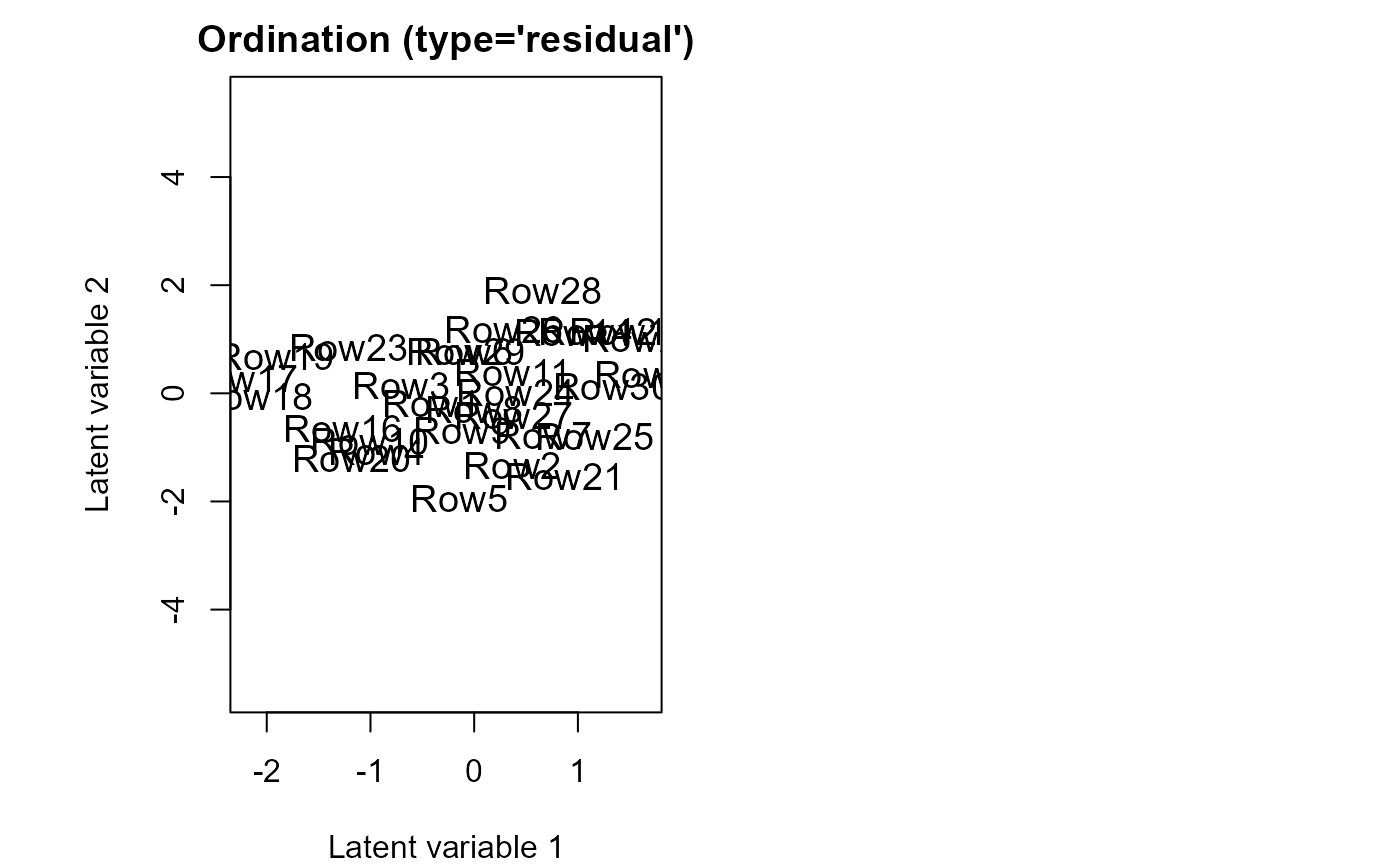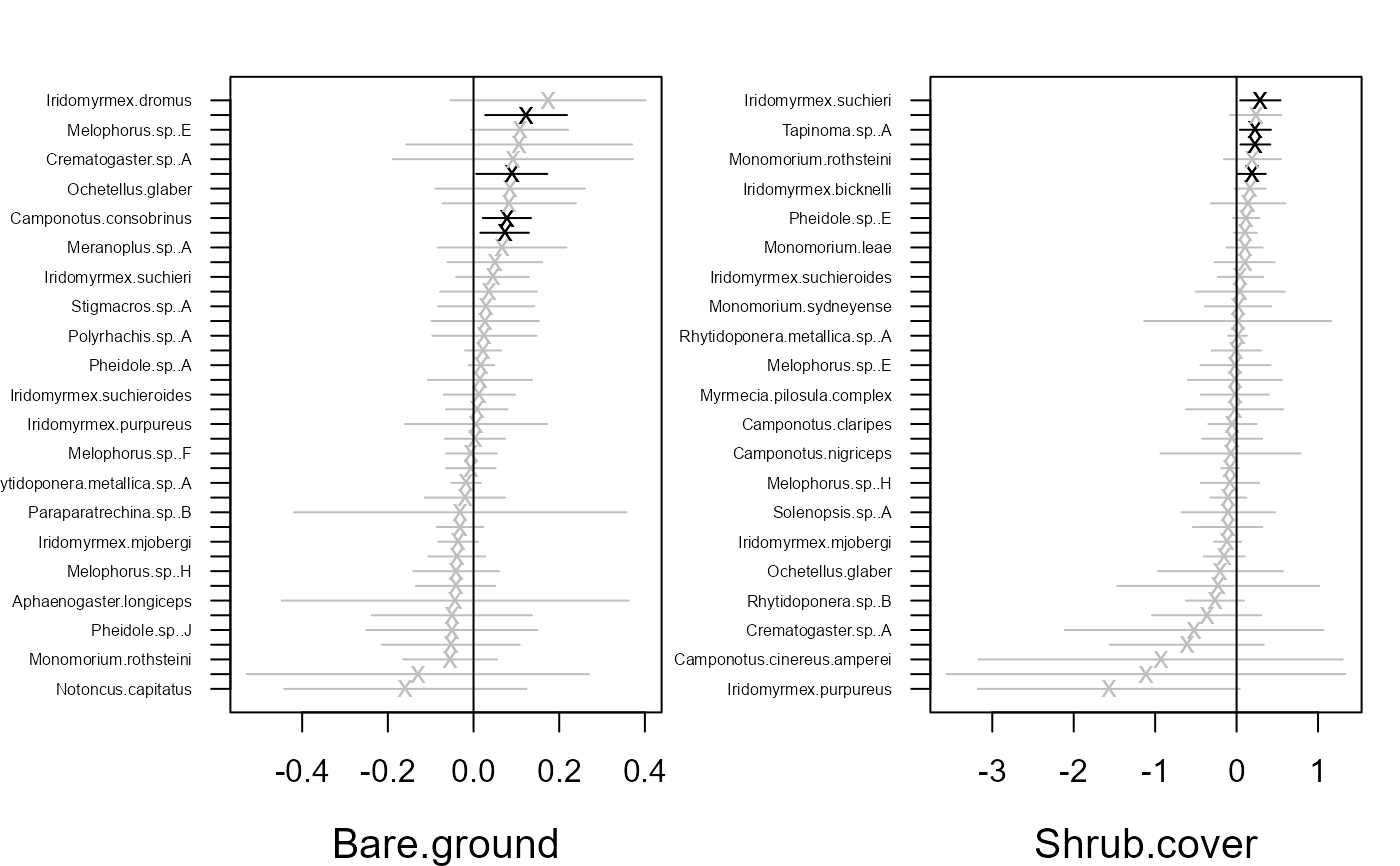## Example 3: Data in long format # Reshape data to long format: datalong <- reshape(data.frame(cbind(y,X)), direction = "long", varying = colnames(y), v.names = "y") head(datalong) #> Bare.ground Canopy.cover Shrub.cover Volume.lying.CWD Feral.mammal.dung #> 1.1 9.00 3.5 0.50 0.03253833 0.20 #> 2.1 6.00 0.0 0.00 0.01202944 0.65 #> 3.1 9.25 0.0 8.10 0.00804195 0.00 #> 4.1 23.25 0.0 0.25 0.00000000 0.20 #> 5.1 8.75 0.0 3.35 0.00993944 0.35 #> 6.1 32.25 0.0 0.00 0.03142221 1.00 #> time y id #> 1.1 1 0 1 #> 2.1 1 0 2 #> 3.1 1 0 3 #> 4.1 1 4 4 #> 5.1 1 2 5 #> 6.1 1 0 6fitvLong <- gllvm(data = datalong, formula = y ~ Bare.ground + Shrub.cover, family = "negative.binomial") ## Example 4: Fourth corner model # Fit fourth corner model with two latent variables fitF1 <- gllvm(y = y, X = X, TR = TR, family = "negative.binomial") coefplot(fitF1)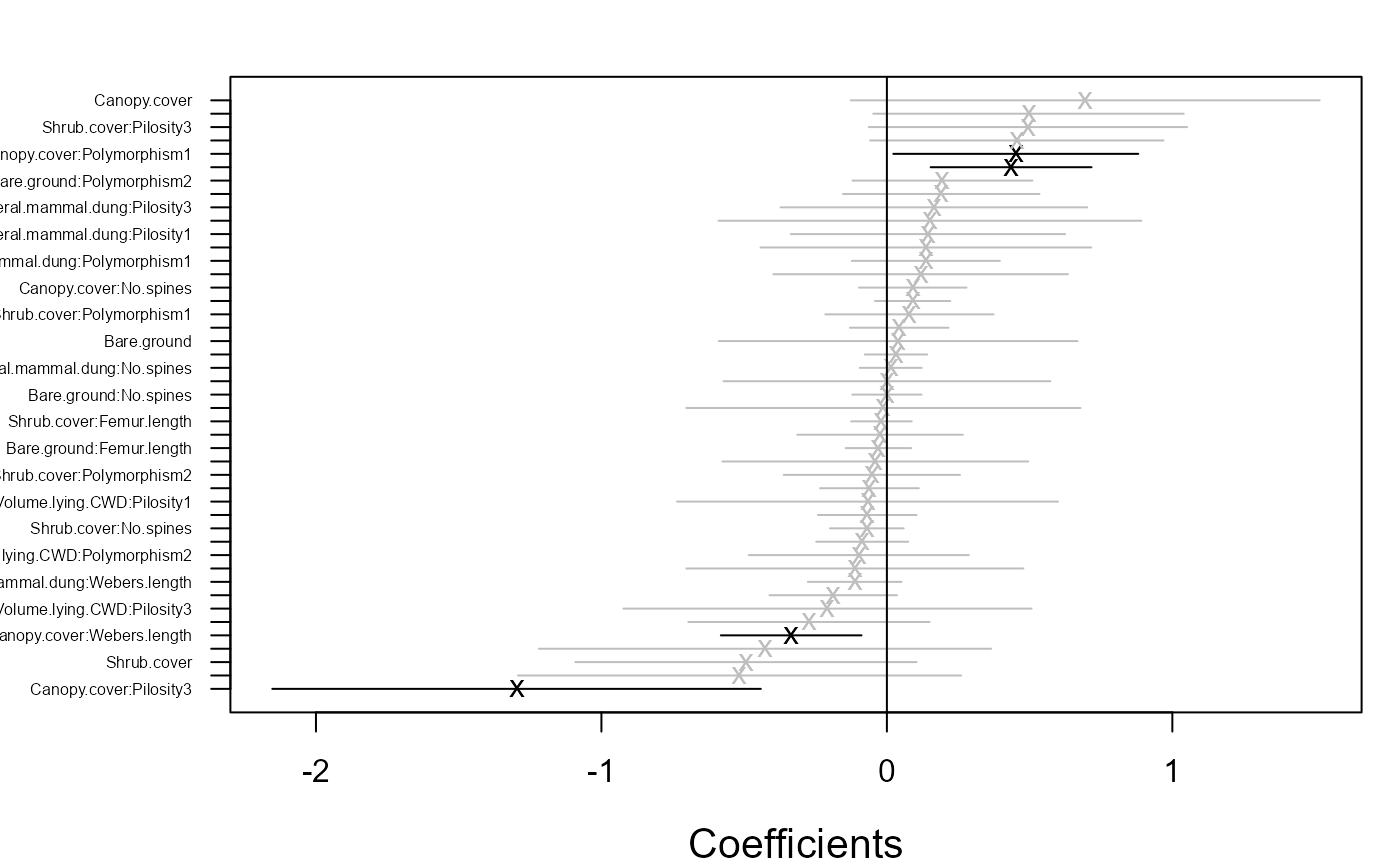# Fourth corner can be plotted also with next lines #fourth = fitF1$fourth.corner
#library(lattice)
#a = max( abs(fourth) )
#colort = colorRampPalette(c("blue","white","red"))
#plot.4th = levelplot(t(as.matrix(fourth)), xlab = "Environmental Variables",
#              ylab = "Species traits", col.regions = colort(100),
#              at = seq( -a, a, length = 100), scales = list( x = list(rot = 45)))
#print(plot.4th)

# Specify model using formula
fitF2 <- gllvm(y = y, X = X, TR = TR,
formula = ~ Bare.ground + Canopy.cover * (Pilosity + Webers.length),
family = "negative.binomial")
ordiplot(fitF2)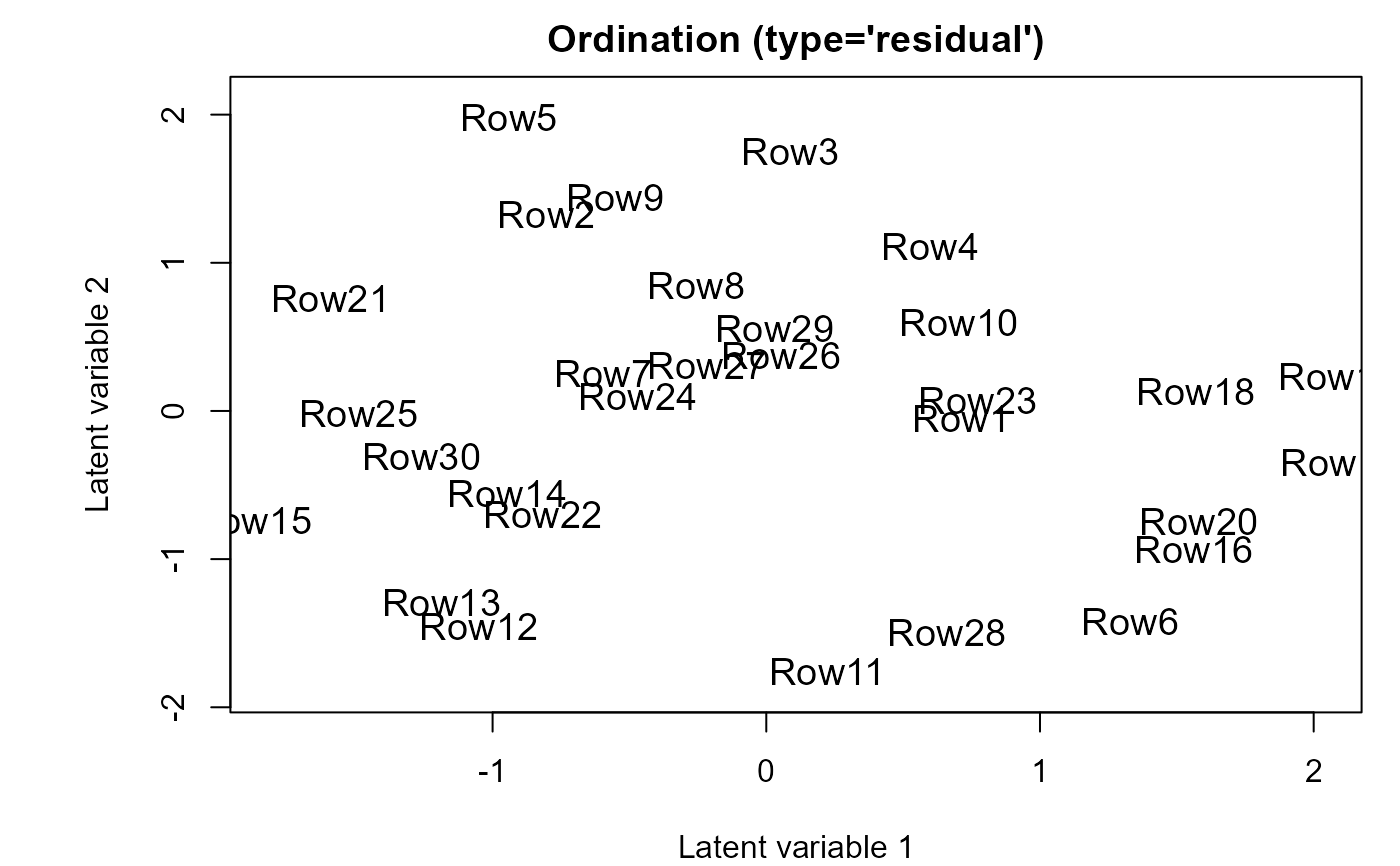coefplot(fitF2)## Include species specific random slopes to the fourth corner model
fitF3 <- gllvm(y = y, X = X, TR = TR,
formula = ~ Bare.ground + Canopy.cover * (Pilosity + Webers.length),
family = "negative.binomial", randomX = ~ Bare.ground + Canopy.cover,
control.start = list(n.init = 3))
ordiplot(fitF3)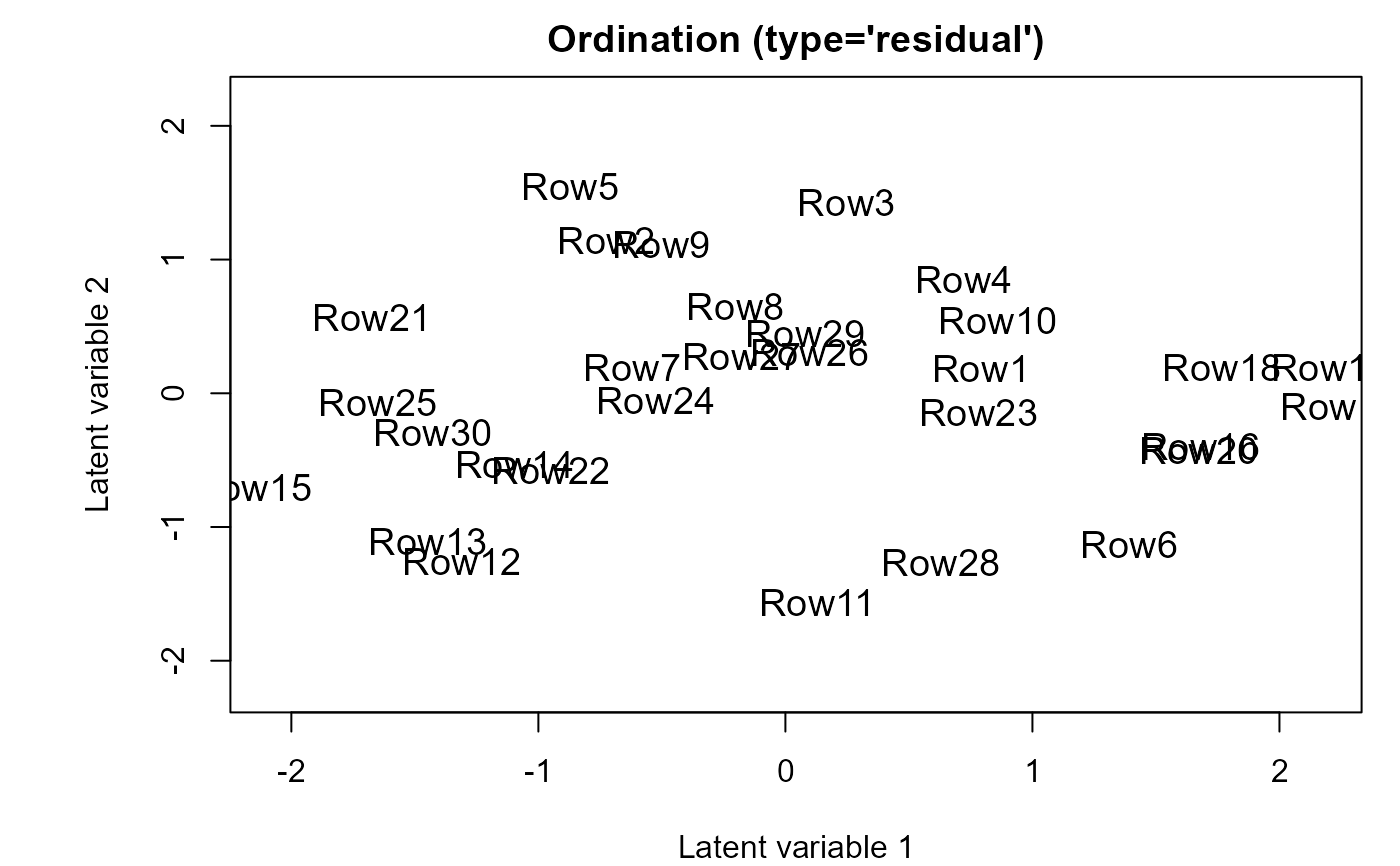coefplot(fitF3)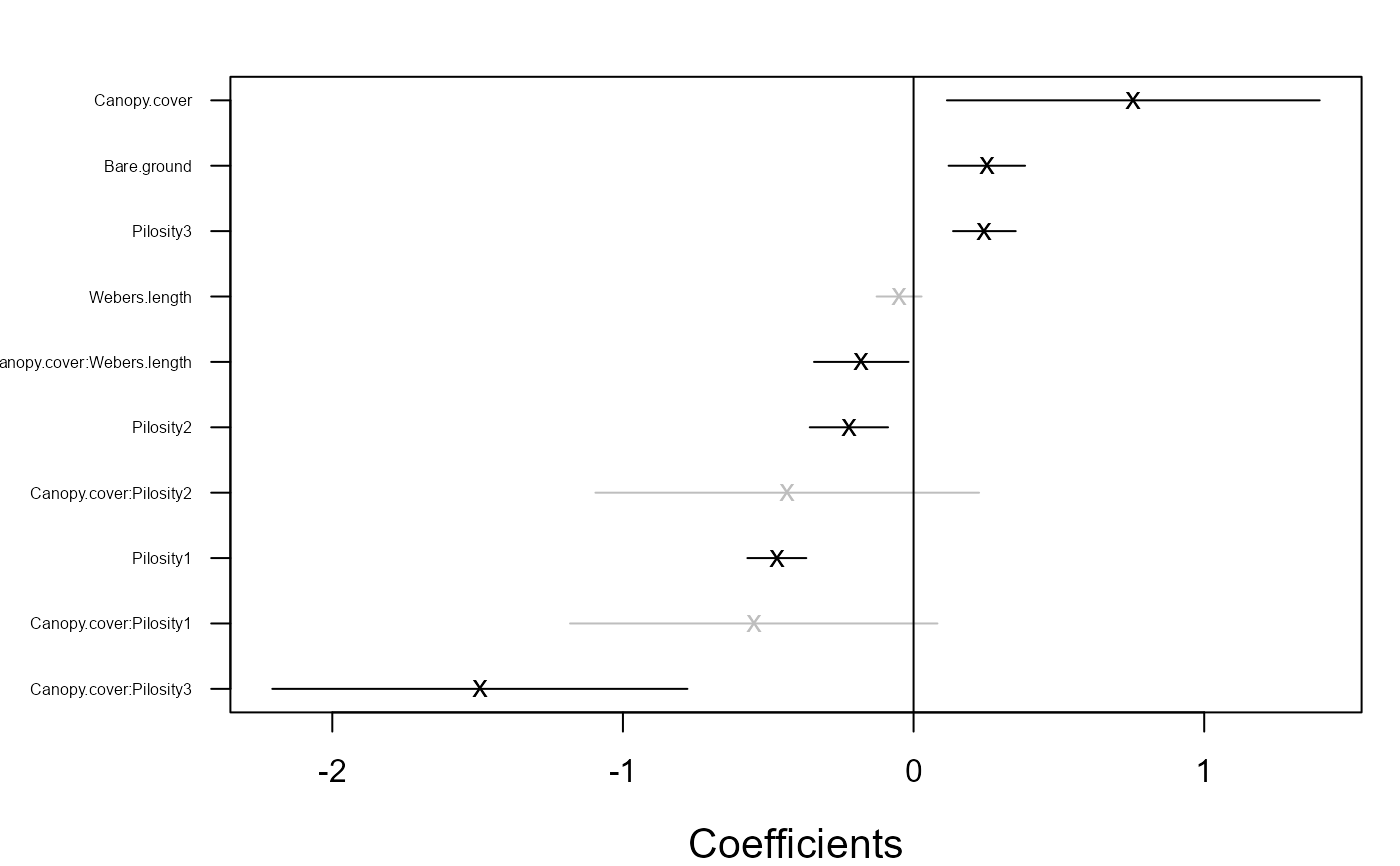## Example 5: Fit Tweedie model
ycoral <- tikus$abund # Let's consider only years 1981 and 1983 ycoral <- ycoral[((tikus$x$time == 81) + (tikus$x\$time == 83)) > 0, ]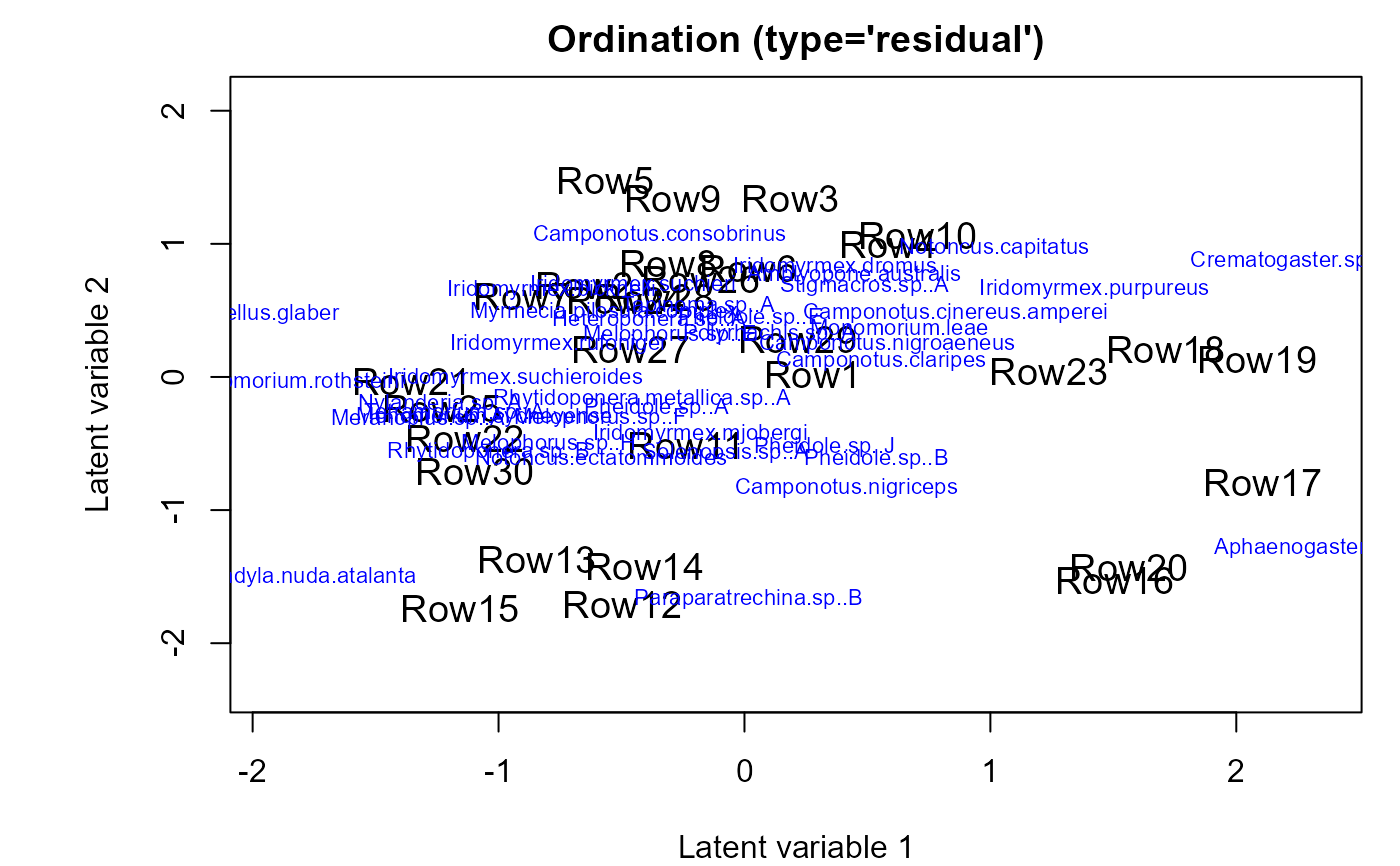# }